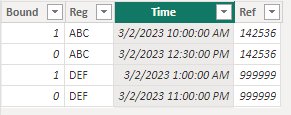cancel
Showing results for
Did you mean:Helper III

## date/time difference between 2 columns if they have the same reference

Hi

I want to calculate the number of HH:MM between 2 columns - both have the same reference however the inbound has 1 and outbound has 0 and same reg too.

e.g.

Bound    Reg            Time                         Ref

1            ABC      03/02/2023 10:00       142536

0            ABC      03/02/2023 12:30       142536

The difference for reg: ABC  or Ref 142536 should be 02:30 (HH:MM)

Thanks.

1 ACCEPTED SOLUTIONCommunity Champion

hi @AI14

Not sure if i fully get you, supposing your data is like:you can plot a table with the ref column and a measure like:

``````Duration =
VAR _reg = MAX(TableName[Reg])
VAR _time1 =
MAXX(
FILTER(
ALL(TableName),
TableName[Reg] = _reg
),
TableName[Time]
)
VAR _time2 =
MINX(
FILTER(
ALL(TableName),
TableName[Reg] = _reg
),
TableName[Time]
)
RETURN
FORMAT(_time2 - _time1, "h:mm")``````

it worked like:4 REPLIES 4Community Champion

hi @AI14

Not sure if i fully get you, supposing your data is like:you can plot a table with the ref column and a measure like:

``````Duration =
VAR _reg = MAX(TableName[Reg])
VAR _time1 =
MAXX(
FILTER(
ALL(TableName),
TableName[Reg] = _reg
),
TableName[Time]
)
VAR _time2 =
MINX(
FILTER(
ALL(TableName),
TableName[Reg] = _reg
),
TableName[Time]
)
RETURN
FORMAT(_time2 - _time1, "h:mm")``````

it worked like:Helper III

Thank you it worked!Super User

Hi @AI14

Where are you planning to show the result? Are you looking for a measure?Please accept the solution when done and consider giving a thumbs up if posts are helpful.  Contact me privately for support with any larger-scale BI needs, tutoring, etc.Helper III

to show on a table and a chart